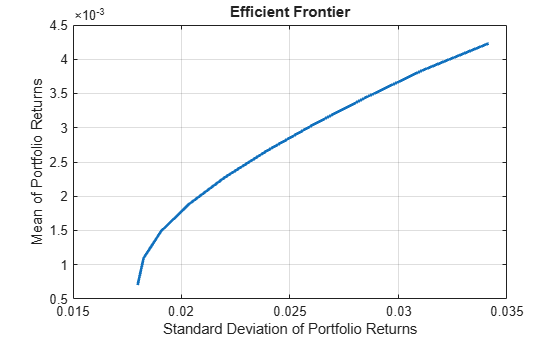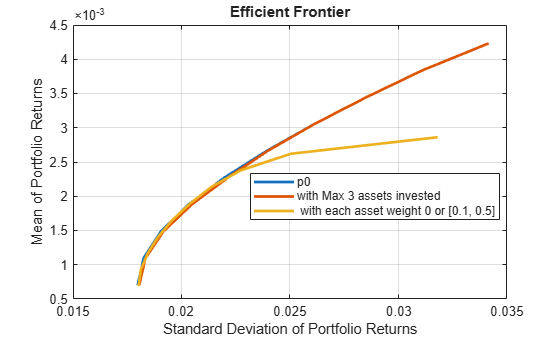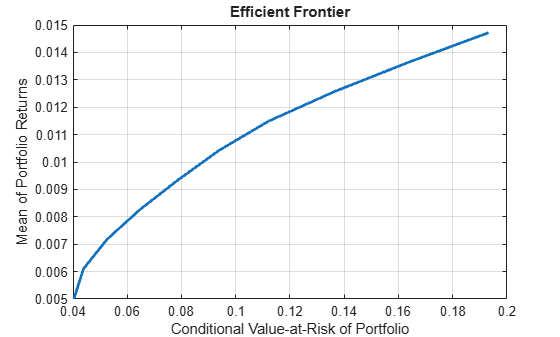# plotFrontier

Plot efficient frontier

## Syntax

``````[prsk,pret] = plotFrontier(obj)``````
``````[prsk,pret] = plotFrontier(obj,NumPorts)``````
``````[prsk,pret] = plotFrontier(obj,PortWeights)``````
``````[prsk,pret] = plotFrontier(obj,PortRisk,PortReturn)``````

## Description

example

``````[prsk,pret] = plotFrontier(obj)``` estimates the efficient frontier with default number of 10 portfolios on the frontier, and plots the corresponding efficient frontier for `Portfolio`, `PortfolioCVaR`, or `PortfolioMAD` objects. For details on the respective workflows when using these different objects, see Portfolio Object Workflow, PortfolioCVaR Object Workflow, and PortfolioMAD Object Workflow.```

example

``````[prsk,pret] = plotFrontier(obj,NumPorts)``` estimates the efficient frontier with a specified number portfolios on the frontier, and plots the corresponding efficient frontier. The number of portfolios is defined by `NumPorts`.```

example

``````[prsk,pret] = plotFrontier(obj,PortWeights)``` estimates efficient portfolio risks and returns with `PortWeights`, and plots the efficient frontier with those portfolios. This syntax assumes that you provide valid efficient portfolio weights as input. `PortWeights` is a `NumAsset`-by-`NumPorts` matrix.```

example

``````[prsk,pret] = plotFrontier(obj,PortRisk,PortReturn)``` plots the efficient frontier with the given risks and returns. This syntax assumes that you provide valid inputs for efficient portfolio risks and returns. `PortRisk` and `PortReturn` are vectors with the same size. Note`plotFrontier` handles multiple input formats as described above. Given an asset universe with `NumAssets` assets and an efficient frontier with `NumPorts` portfolios, remember that portfolio weights are `NumAsset`-by-`NumPorts` matrices and that portfolio risks and returns are `NumPorts` column vectors. ```

## Examples

collapse all

Given a portfolio `p`, plot the efficient frontier.

```load CAPMuniverse p = Portfolio('AssetList',Assets(1:12)); p = estimateAssetMoments(p, Data(:,1:12),'missingdata',true); p = setDefaultConstraints(p); plotFrontier(p);```Create a `Portfolio` object for 12 stocks based on `CAPMuniverse.mat`.` `

```load CAPMuniverse p0 = Portfolio('AssetList',Assets(1:12)); p0 = estimateAssetMoments(p0, Data(:,1:12),'missingdata',true); p0 = setDefaultConstraints(p0);```

Use `setMinMaxNumAssets` to define a maximum number of 3 assets.

`pWithMaxNumAssets = setMinMaxNumAssets(p0, [], 3);`

Use `setBounds` to define a lower and upper bound and a `BoundType` of `'Conditional'`.

`pWithConditionalBound = setBounds(p0, 0.1, 0.5,'BoundType', 'Conditional');`

Use `plotFrontier` to compare the different portfolio objects.

```figure; plotFrontier(p0); hold on; plotFrontier(pWithMaxNumAssets); hold on; plotFrontier(pWithConditionalBound); hold off; legend('p0', 'with Max 3 assets invested', ' with each asset weight 0 or [0.1, 0.5]', 'location', 'best');```Define a target return and use `estimateFrontierByReturn` to compare the three portfolio objects.

```targetRetn = 2.0e-3; pwgt0 = estimateFrontierByReturn(p0, targetRetn); pwgtWithMaxNumAssets = estimateFrontierByReturn(pWithMaxNumAssets, targetRetn); pwgtConditionalBound = estimateFrontierByReturn(pWithConditionalBound, targetRetn); ```

The following table shows the final allocation for specified target return among the three portfolio objects. You can see that the small positions in `'AAPL'`and `'HPQ'` are avoided in `pwgtConditionalBound`, and only three assets are invested in `pwgtWithMaxNumAssets`.

`result = table(p0.AssetList',pwgt0,pwgtWithMaxNumAssets,pwgtConditionalBound)`
```result=12×4 table Var1 pwgt0 pwgtWithMaxNumAssets pwgtConditionalBound ________ ________ ____________________ ____________________ {'AAPL'} 0.076791 0 0.1 {'AMZN'} 0 0 0 {'CSCO'} 0 0 0 {'DELL'} 0 0 0 {'EBAY'} 0 0 0 {'GOOG'} 0.44841 0.47297 0.44255 {'HPQ' } 0.022406 -8.3267e-17 0 {'IBM' } 0.31139 0.34763 0.31592 {'INTC'} 0 0 0 {'MSFT'} 0.14101 0.1794 0.14153 {'ORCL'} 0 0 0 {'YHOO'} 0 0 0 ```

Given a PortfolioCVaR `p`, plot the efficient frontier.

```m = [ 0.05; 0.1; 0.12; 0.18 ]; C = [ 0.0064 0.00408 0.00192 0; 0.00408 0.0289 0.0204 0.0119; 0.00192 0.0204 0.0576 0.0336; 0 0.0119 0.0336 0.1225 ]; m = m/12; C = C/12; AssetScenarios = mvnrnd(m, C, 20000); p = PortfolioCVaR; p = setScenarios(p, AssetScenarios); p = setDefaultConstraints(p); p = setProbabilityLevel(p, 0.95); plotFrontier(p);```Given a PortfolioMAD `p`, plot the efficient frontier.

```m = [ 0.05; 0.1; 0.12; 0.18 ]; C = [ 0.0064 0.00408 0.00192 0; 0.00408 0.0289 0.0204 0.0119; 0.00192 0.0204 0.0576 0.0336; 0 0.0119 0.0336 0.1225 ]; m = m/12; C = C/12; AssetScenarios = mvnrnd(m, C, 20000); p = PortfolioMAD; p = setScenarios(p, AssetScenarios); p = setDefaultConstraints(p); plotFrontier(p);```## Input Arguments

collapse all

Object for portfolio, specified using `Portfolio`, `PortfolioCVaR`, or `PortfolioMAD` object. For more information on creating a portfolio object, see

Data Types: `object`

Number of points to obtain on the efficient frontier, specified as a scalar integer.

Note

If no value is specified for `NumPorts`, the default value is obtained from the hidden property `defaultNumPorts` (default value is `10`). If `NumPorts` = `1`, this function returns the portfolio specified by the hidden property `defaultFrontierLimit` (current default value is `'min'`).

Data Types: `double`

Standard deviations of portfolio returns for each portfolio, specified as a vector.

Note

`PortRisk` and `PortReturn` must be vectors with the same size.

Data Types: `double`

Means of portfolio returns for each portfolio, specified as a vector.

Note

`PortRisk` and `PortReturn` must be vectors with the same size.

Data Types: `double`

Optimal portfolios on the efficient frontier, specified as a `NumAsset`-by-`NumPorts` matrix.

Data Types: `double`

## Output Arguments

collapse all

Estimated efficient portfolio risks (standard deviation of returns, returned as a vector for a `Portfolio`, `PortfolioCVaR`, or `PortfolioMAD` input object (`obj`).

Note

• If the portfolio object has a name in the `Name` property, the name is displayed as the title of the plot. Otherwise, the plot is labeled “Efficient Frontier.”

• If the portfolio object has an initial portfolio in the `InitPort` property, the initial portfolio is plotted and labeled.

• If portfolio risks and returns are inputs, make sure that risks come first in the calling sequence. In addition, if portfolio risks and returns are not sorted in ascending order, this method performs the sort. On output, the sorted moments are returned.

Estimated efficient portfolio returns, returned as a vector for a `Portfolio`, `PortfolioCVaR`, or `PortfolioMAD` input object (`obj`).

Note

• If the portfolio object has a name in the `Name` property, the name is displayed as the title of the plot. Otherwise, the plot is labeled “Efficient Frontier.”

• If the portfolio object has an initial portfolio in the `InitPort` property, the initial portfolio is plotted and labeled.

• If portfolio risks and returns are inputs, make sure that risks come first in the calling sequence. In addition, if portfolio risks and returns are not sorted in ascending order, this method performs the sort. On output, the sorted moments are returned.

## Tips

You can also use dot notation to plot the efficient frontier.

`[prsk, pret] = obj.plotFrontier;`

## Version History

Introduced in R2011a